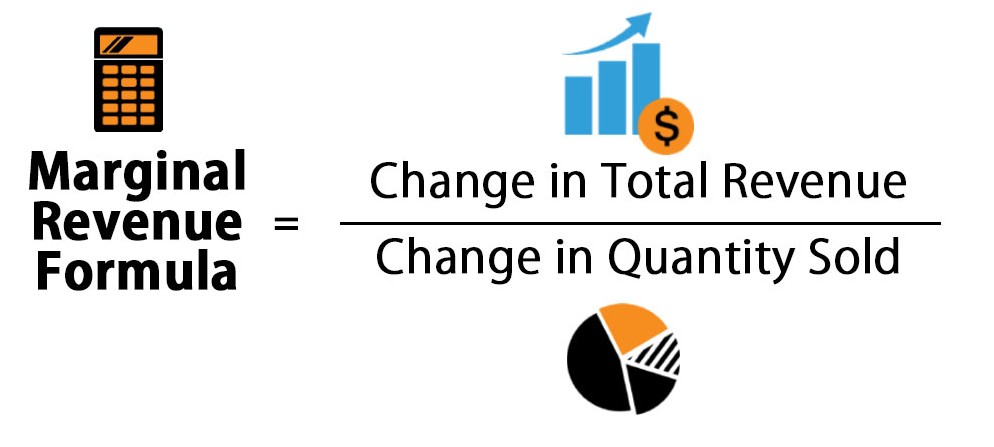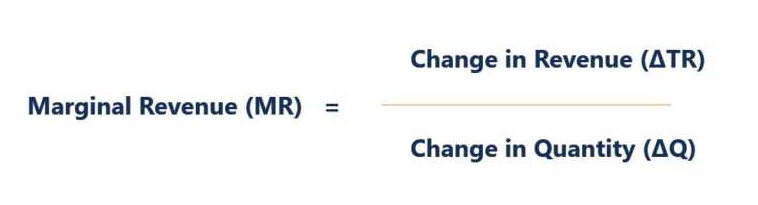# ,How To Calculate Marginal Revenue?

Marginal Revenue is easy to calculate.  All you need to remember is that marginal revenue is the revenue obtained from the additional unit sold. The formula above breaks into two parts: one, change in revenue that means (total revenue – old revenue) and two, change in quantity, which means (total quantity – old quantity).

A firm’s profits will be maximized when marginal revenue (MR) equals marginal cost (MC). If {\displaystyle MR>MC}then a profit-maximizing firm will increase output for more profit, while if {\displaystyle MR<MC}then the firm will decrease output for additional profit. Thus the firm will choose the profit-maximizing output level as that for which {\displaystyle MR=MC}.

Example: Mr. A used to sell 10 pencils and now he is selling 15. Earlier, his total revenue was $20 and it is now$28. Putting the values in the formula change in revenue = $8 and change in quantity = 5 pieces, so,$8/5 = $1.60, which is his marginal revenue per additional unit sold.## Marginal Revenue marginal revenue (MR) is the additional revenue that will be generated by increasing product sales by one unit. In a perfectly competitive market, the additional revenue generated by selling an additional unit of a good is equal to the price the firm is able to charge the buyer of the good. This is because a firm in a competitive market will always get the same price for every unit it sells regardless of the number of units the firm sells since the firm’s sales can never impact the industry’s price. However, a monopoly determines the entire industry’s sales. As a result, it will have to lower the price of all units sold to increase sales by 1 unit. Therefore, the marginal revenue generated is always lower than the price the firm is able to charge for the unit sold, since each reduction in price causes unit revenue to decline on every good the firm sells. The marginal revenue (the increase in total revenue) is the price the firm gets on the additional unit sold, less the revenue lost by reducing the price on all other units that were sold prior to the decrease in price. ## Marginal Revenue Formula Marginal Revenue (MR) is the increase in the Total Revenue (TR) that is gained when the firm sells one additional (marginal) unit of that product. In other words, MR is the revenue obtained from the last unit sold. Marginal Revenue can remain uniform at a particular level of output. However, the MR will eventually slow down as the production level rises due to the Law of Diminishing Returns. The Law of Diminishing Returns refers to a point at which the level of benefits gained is less than the amount of effort invested. For example, we assume that when a firm sells 5, 6 and seven units of a good, the firm’s Total Revenue is$20, $25 and$28 respectively. Therefore, when the quantity sold is 5, the firm’s MR from selling the new unit or the 6th unit will be $25 –$20 = $5.## Marginal Revenue Product As you can see from our example, the marginal revenue definition is a pretty simply concept. It does, however, have a huge influence over product pricing and production levels based on the manufacturer’s industry and product. For instance, in a truly competitive market place where manufacturers are selling mass-produced, homogenous products at the market price, the marginal revenue is equal to the market price. In other words, manufacturers of commodities with little differentiation will always sell their products at the market price because it’s a competitive market place. If they raise their prices, consumers will buy from one of their competitors. You can think this as a farmer who sells corn. The market sets the corn price each year. If he charges more than the market, consumers will purchase corn from his competitors because there is no difference between his product and theirs. The opposite is true in a low output or highly specialized industry. Since there are fewer product alternatives available, the production level of the company affects the selling price. In other words, less supply will increase demand and increase the willingness of consumers to pay higher prices. The company obviously has to keep the marginal revenue product inside the constraints of the price elasticity curve, but they can adjust their output and pricing structure to optimize their profitability. ## How To Find Marginal Revenue Jan’s Machining is a manufacturer of office supplies. Jan is currently focused on the upcoming production run of specialty pencils. Using the margin revenue curve to figure out how much to produce and set the sales price. Jan operates in an industry with several limited competitors and a set demand. Jan figures that she can produce 100 pencils and sell them for$150 each resulting in $15,000 of revenues. Continuing with her analysis, Jan estimates that she will need to drop the price from$150 a pencil to $149 a pencil if she produces more than 2,000 units. Here’s how to find marginal revenue if Jan produced one extra unit. Since Jan had to drop her price$1 in order to produce and sell an extra unit, her revenue per unit went down, but her total revenues went up. Thus, Jan’s marginal revenue for this product is $49. We calculated that by multiplying the new production amount (2,001 units) by the new price ($149) and subtracting the original revenue number (2,000 units x $150 =$15,000).

This example can be expanded into different products, quantities, and industries, but we will keep it simple for now.

## What Is Marginal Revenue

economic concept analyzes the profitability of selling more products. The purpose of this calculation is to perform some comparisons in order to evaluate a decision of increasing the number of units being sold. The MR should be compared with the current price per unit or against the marginal cost of producing one more unit.

If the MR is equal to the price it means that in order to sale more units the company doesn’t have to change its price. This is not normally the case in practice because selling one additional unit is not a real life situation but this concept can also be applied to ranges of hundred units or even thousands. The MR should be compared with Marginal Cost and as long as the MR stays higher it will be profitable for the company to produce and sell an additional unit.

## Marginal Revenue Calculator

Movement Bicycles LLC is a company that produces bicycles for professional athletes. The company manager, Mr. Chen wants to analyze the possibility of increasing the company’s production by 10%. Currently, Movement Bicycles produces 100 bicycles per month, so the goal will be to produce 110 units per month. Mr. Chen wants to calculate the profitability of doing this. Can he use MR to evaluate the profitability of this new scenario?

The answer is yes. By calculating the marginal revenue of this new production level Mr. Chen can then go ahead and compare it with the marginal cost of producing those 10 additional units and if the marginal revenue is higher than the marginal cost then the new set up will be profitable for the company.Marginal Revenue Curve.

Let us examine the concept of Marginal Revenue in greater detail. The Marginal Cost curve is a “U” shape curve because the marginal cost for 1-5 additional units will be less and with more incremental units, the marginal cost will begin to rise.

The Marginal Revenue curve is sloping downwards because, with one additional unit sold, we would generate revenue close to our normal revenue but as we start selling more and more, we would require reducing the price of the item we are selling. Otherwise, we will not be able to sell it, which is also known as the law of diminishing margin. So, the more you sell after a normal limit, the more the price will diminish and so will the revenue.

## Marginal Revenue Definition

Marginal revenue is equal to the ratio of the change in revenue for some change in quantity sold to that change in quantity sold. This can also be represented as a derivative when the change in quantity sold becomes arbitrarily small. Define the revenue function to be

{\displaystyle R(Q)=P(Q)\cdot Q,}

where Q is output and P(Q) is the inverse demand function of customers. By the product rule, marginal revenue is then given by

{\displaystyle R'(Q)=P(Q)+P'(Q)\cdot Q,}

where the prime sign indicates a derivative. For a firm facing perfect competition, price does not change with quantity sold ({\displaystyle P'(Q)=0}), so marginal revenue is equal to price. For a monopoly, the price decreases with quantity sold ({\displaystyle P'(Q)<0}), so marginal revenue is less than price (for positive {\displaystyle Q.})

## Marginal Revenue Equation

##### TR = P * Q

Marginal revenue is another important measure. Revenue obtained from the last unit sold. This is computed by taking the change in total revenue divided by the change in quantity.

##### MR = Change in TR / Change in Q

For competitive firms, marginal revenue isn’t very interesting. If all units are sold for the market price, then marginal revenue will simply be the market price. In the table below, you can see that the marginal revenue is constant for all cakes sold–$6. From that information, and by remembering that we are talking about a competitive market. We can easily tell that the market price for cakes is$6. So the last cake will be sold for $6 as long as the market price remains constant. Competitive firms have a constant MR curve. A cake sold for$6 is \$6 of additional revenue.

## Marginal Revenue Product Formula

Marginal Revenue (MR) of the firm at any quantity of output sold is the increment in its total revenue (TR). When the firm sells the marginal (or the additional) unit of that quantity. In other words, marginal revenue at any output (q) is the (extra) revenue. That the firm obtains from selling the marginal (or the additional) unit of that output.

Suppose, when the quantities sold (q) are 9, 10 and 11 units. The firm’s TRs are, 50, 55 and 58 (Rs) respectively. Here, at q = 10, the firm’s MR obtained from selling the marginal unit. Or the 10th unit = 55 – 50 = 5 (Rs). Similarly, at q = 11, MR = 58 — 55 = 3 (Rs).